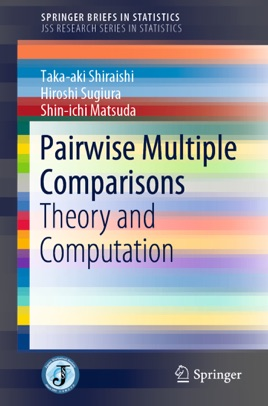• 42,99 €

## Publisher Description

This book focuses on all-pairwise multiple comparisons of means in multi-sample models, introducing closed testing procedures based on maximum absolute values of some two-sample t-test statistics and on F-test statistics in homoscedastic multi-sample models. It shows that (1) the multi-step procedures are more powerful than single-step procedures and the Ryan/Einot–Gabriel/Welsh tests, and (2) the confidence regions induced by the multi-step procedures are equivalent to simultaneous confidence intervals. Next, it describes the multi-step test procedure in heteroscedastic multi-sample models, which is superior to the single-step Games–Howell procedure. In the context of simple ordered restrictions of means, the authors also discuss closed testing procedures based on maximum values of two-sample one-sided t-test statistics and based on Bartholomew's statistics. Furthermore, the book presents distribution-free procedures and describes simulation studies performed under the null hypothesis and some alternative hypotheses. Although single-step multiple comparison procedures are generally used, the closed testing procedures described are more powerful than the single-step procedures. In order to execute the multiple comparison procedures, the upper 100α percentiles of the complicated distributions are required. Classical integral formulas such as Simpson's rule and the Gaussian rule have been used for the calculation of the integral transform that appears in statistical calculations. However, these formulas are not effective for the complicated distribution. As such, the authors introduce the sinc method, which is optimal in terms of accuracy and computational cost.